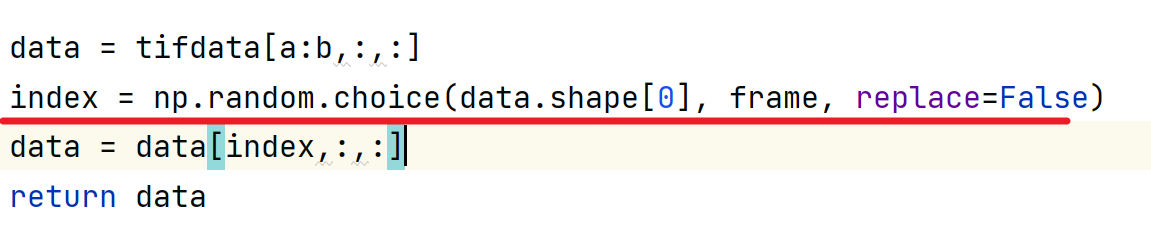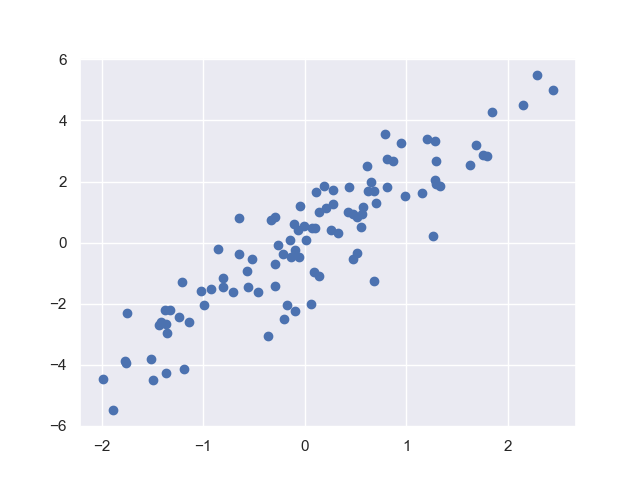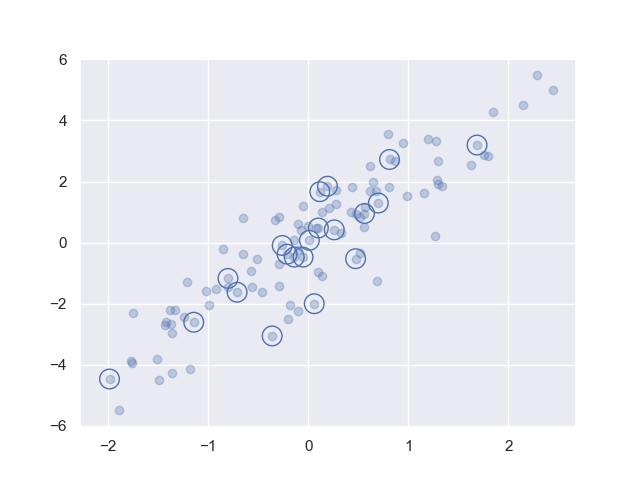• python中的numpy数组中数据提取办法
2022-03-17 13:52:46

1、如果在numpy中使用shape方法，得到的数组的尺寸为（n,），则表示数组只有一列，则取原则，只需要使用a[k]即可，如果尺寸为(n,1)，如果取一个元素，则需要使用a[0,k]这种方式python
更多相关内容
• 目录Numpy数组中数据的抽取1.比较操作2.操作布尔数组统计个数记录True的个数numpy.count_nonzero函数记录True的个数numpy.sum函数快速查阅3.将布尔数组作为掩码4.花哨的索引单纯的花哨索引组合索引花哨索引与普通...
•展开全文• 排序numpy的排序函数sort函数返回排序后的数组lexsort函数根据键值的字典序进行排序argsort函数返回数组排序后的下标ndarray类的sort方法可以对数组进行原地排序msort函数沿着第一个轴排序sort_complex函数对复数...

排序

numpy的排序函数

sort函数返回排序后的数组

lexsort函数根据键值的字典序进行排序

argsort函数返回数组排序后的下标

ndarray类的sort方法可以对数组进行原地排序

msort函数沿着第一个轴排序

sort_complex函数对复数按照先实部后虚部的顺序进行排序

Key_Function

lexsort函数根据键值的字典序进行排序

将两个数组构成的元组, 分别取出对应下标的元素, 第一个数组的看做key, 第二个数组看做value

然后按照value进行排序, 返回排序后的数组下标

字典序就是按value进行排序

Code

importnumpy as npimportdatetimedefdatestr2num(s):

s= s.decode("utf-8")return datetime.datetime.strptime(s, "%d-%m-%Y").toordinal()

dates, closes= np.loadtxt("AAPL.csv", delimiter=',', usecols=(1, 5),

converters={1:datestr2num}, unpack=True)

indices=np.lexsort((dates, closes))print(indices)'''[ 0 16 1 17 18 4 3 2 5 28 19 21 15 6 29 22 27 20 9 7 25 26 10 8 14

11 23 12 24 13]'''l=[(datetime.date.fromordinal(int(dates[i])), closes[i]) for i inindices]print(l)'''[(datetime.date(2011, 1, 28), 336.10000000000002),

(datetime.date(2011, 2, 22), 338.61000000000001),

(datetime.date(2011, 1, 31), 339.31999999999999),

(datetime.date(2011, 2, 23), 342.62),

(datetime.date(2011, 2, 24), 342.88),

(datetime.date(2011, 2, 3), 343.44),

(datetime.date(2011, 2, 2), 344.31999999999999),

(datetime.date(2011, 2, 1), 345.02999999999997),

(datetime.date(2011, 2, 4), 346.5),

(datetime.date(2011, 3, 10), 346.67000000000002),

(datetime.date(2011, 2, 25), 348.16000000000003),

(datetime.date(2011, 3, 1), 349.31),

(datetime.date(2011, 2, 18), 350.56),

(datetime.date(2011, 2, 7), 351.88),

(datetime.date(2011, 3, 11), 351.99000000000001),

(datetime.date(2011, 3, 2), 352.12),

(datetime.date(2011, 3, 9), 352.47000000000003),

(datetime.date(2011, 2, 28), 353.20999999999998),

(datetime.date(2011, 2, 10), 354.54000000000002),

(datetime.date(2011, 2, 8), 355.19999999999999),

(datetime.date(2011, 3, 7), 355.36000000000001),

(datetime.date(2011, 3, 8), 355.75999999999999),

(datetime.date(2011, 2, 11), 356.85000000000002),

(datetime.date(2011, 2, 9), 358.16000000000003),

(datetime.date(2011, 2, 17), 358.30000000000001),

(datetime.date(2011, 2, 14), 359.18000000000001),

(datetime.date(2011, 3, 3), 359.56),

(datetime.date(2011, 2, 15), 359.89999999999998),

(datetime.date(2011, 3, 4), 360.0),

(datetime.date(2011, 2, 16), 363.13)]'''

对复数进行排序

复数包含实数部分和虚数部分

NumPy中有复数类型, 使用两个浮点数来表示复数.

Key_Function

sort_complex函数对复数进行排序, 按照先实部后虚部的顺序排序

Code

importnumpy as np

np.random.seed(42)#随机数种子, 使用随机数种子, 不管参数如何, 都会生成固定顺序的随机数数组#参数只是起始随机数在随机数数组中的位置#同一个随机数种子, 反复取随机数, 肯定结果是不同的#可能因为所选取的随机数在随机数组中下标在递增

complex_numbers = np.random.random(5) + 1j * np.random.random(5)print(complex_numbers)'''[ 0.37454012+0.15599452j 0.95071431+0.05808361j 0.73199394+0.86617615j

0.59865848+0.60111501j 0.15601864+0.70807258j]'''

print(np.sort_complex(complex_numbers))'''[ 0.15601864+0.70807258j 0.37454012+0.15599452j 0.59865848+0.60111501j

0.73199394+0.86617615j 0.95071431+0.05808361j]'''

numpy数组中搜索

argmax函数返回数组中最大值对应的下标

a = np.array([2, 4, 8])

np.argmax(a)#2

nanargmax函数, 会忽略NaN值

b = np.array([np.nan, 2, 4])

np.nanargmax(b)#2

argmin和nanargmin函数, 返回数组中最小值对应的下标

argwhere函数根据搜索条件搜索非零的元素, 并分组返回对应的下标

a = np.array([2, 4, 8])

np.argwhere(a<= 4)'''[

]'''

searchsort函数可以为指定的插入值寻找维持数组排序的索引位置

extract函数返回满足指定条件的数组元素

Key_Function

searchsorted函数, 返回一个索引位置, 将元素插入这个位置的话, 不改变原数组的有序性

Code

importnumpy as np

a= np.arange(5)print(a)#[0 1 2 3 4]

indices = np.searchsorted(a, [-2, 7])print(indices)#[0 5]

print(np.insert(a, indices, [-2, 7]))#[-2 0 1 2 3 4 7]

numpy中数组元素抽取

Key_Function

extract函数可以根据某个条件从数组中抽取元素.

这个函数和where函数相似

nonzero函数专门用来抽取非零的数组元素

Code

importnumpy as np

a= np.arange(7)print(a)#[0 1 2 3 4 5 6]

condition = (a % 2) ==0print(condition)#[ True False True False True False True]

print(np.extract(condition, a)) #指定了布尔条件从数组中抽取偶数元素#[0 2 4 6]

print(np.nonzero(a))#(array([1, 2, 3, 4, 5, 6]),)

展开全文• 目录Numpy数组中数据的抽取1.比较操作2.操作布尔数组统计个数记录True的个数numpy.count_nonzero函数记录True的个数numpy.sum函数快速查阅3.将布尔数组作为掩码4.花哨的索引单纯的花哨索引组合索引花哨索引与普通...

# Numpy数组中数据的抽取

前面讲解了Numpy中数组的创建,操作,运算等内容

有些时候我们还想要抽取和查看Numpy数组中符合某些条件的值

还有的时候,我们想要统计数组中有多少值大于某一个给定的值,或则删除所有超过设定的阈值的异常点

因此,本章将讲解如下内容:

• 比较,掩码和布尔逻辑
• 高级索引

## 1.比较操作

前面讲解的通用函数,主要关注于加减乘除等算数运算符和其他一些运算符实现了数组的逐元素操作

Numpy中还实现了如<(小于)和>(大于)等逐元素比较的通用函数.

比较运算的结果是一个布尔数据类型的数组.

主要的比较操作有以下几种:

运算符对应通用函数作用
==np.equal比较是否相等
!=np.not_equal比较是否不相等
<np.less比较是否小于
<=np.less_equal比较是否小于等于
>np.greater比较是否大于
>=np.greater_equal比较是否大于等于

下面讲举几个例子:

array_1=np.arange(6)
print(array_1<3)
print(array_1>3)
print(array_1<=3)
print(array_1>=3)
print(array_1!=3)
print(array_1==3)
>>>
[ True  True  True False False False]
[False False False False  True  True]
[ True  True  True  True False False]
[False False False  True  True  True]
[ True  True  True False  True  True]
[False False False  True False False]


此外,我们还可以将比较运算符和普通运算符进行复合运算

print(array_1**2==2**array_1)
>>>
[False False  True False  True False]


其实使用这些比较运算符(封装器)的时候,可以直接调用函数

print(np.equal(array_1**2,2**array_1))
>>>
[False False  True False  True False]


这些比较通用函数还能用于更高维度数组

array_1=np.random.randint(0,10(10,3))
print(array_1<=5)
>>>
[[False  True  True]
[ True  True  True]
[False False  True]
[ True  True False]
[ True  True False]
[ True False  True]
[ True  True  True]
[ True False  True]
[ True  True  True]
[False False  True]]


## 2.操作布尔数组

向上面一样,如果我们得到了布尔数组,我们就可以实现很多有用的操作.

下面就将来讲解这些操作:

### 统计个数

#### 记录True的个数numpy.count_nonzero函数

Numpy.count_nonzero函数主要用于统计一个数组中有多少个True

np.count_nonzero(布尔数组名)


例如:

array_1=np.arange(1,10).reshape(3,3)
print(np.count_nonzero(array_1<3))
>>>
2


#### 记录True的个数numpy.sum函数

由于在Python中,逻辑值False具有数值0,True具有逻辑值1,均可以参与运算.

因此我们可以用numpy.sum函数来计算有多少个True

np.sum(布尔数组名)


此外,如果我们想要求出False的个数,直接用元素个数减去True的个数即可

### 快速查阅

我们在前面的通用函数中,提到了两个函数np.all和np.any

这两个函数用于查询数组中是否全部的值都是某一个值,或者数组是否具有某一个值

这两个函数的参数都要放入布尔数组,然后根据调用的函数,判断数组中是否有True或者是否全是True

np.any(布尔数组)
np.all(布尔数组)


其实一般我们不会放入布尔数组,而是结合判断运算符,来使用

例如:

array_1=np.arange(10)
print(np.any(array_1==6))
print(np.all(array_1<3))
>>>
True
False


此外,我们还能指定沿特定的坐标轴方向,利用axis参数

array_1=np.random.randint((10,3))
print(array_1<5,axis=0)
print(array_1<5,axis=1)
>>>
[ True  True  True]
[ True  True  True  True  True  True  True  True  True  True]


其中:

• axis=0表示沿列查询,axis=1表示沿行查询,最后都会得到一个行向量

## 3.将布尔数组作为掩码

上面我们讲解了如何直接对布尔数组进行聚合计算.
除此以外,我们还能够使用布尔数组作为掩码,通过掩码选择数据的子数据集

掩码用于从数组中抽出特定的数据.

例如:
有一个数组,我们想要从中选出小于5的数字

array_1=np.arange(11)
>>>
[0,1,2,3,4,5,6,7,8,9,10]


我们想要小于5的数字,那么我们可以用另外一个"数组"来结合

[0  ,1  ,2 ,3  ,4  ,5   ,6   ,7    ,8   ,9   ,10]
[要 ,要 ,要 ,要 ,要 ,不要 ,不要 ,不要 ,不要 ,不要 ,不要]


这样结合之后,我们就能够得到我们需要的数字.

这样,第二个数组就是掩码,用于掩盖我们不需要的数字

Numpy中的布尔数组可以直接用做索引,用做掩码操作

数组[布尔数组]


例如:

array_1=np.arange(10)
print(array_1[array_1<5])
>>>
[0 1 2 3 4]


通过这种方法,最后返回的是所有符合要求的值的一个向量

array_1=np.arange(9).reshape(3,3)
print(array_1[array_1<6])
>>>
[0 1 2 3 4 5]


## 4.花哨的索引

前面我们曾经讲解过用偏移量来获取单个值或者用切片来获取多个值.

其实Numpy中还支持更加强大的索引操作,称为花哨的索引(fancy indexing)

它支持传入索引数组,因此花哨的索引能够实现更加强大的功能.下面就将来讲解花哨的索引

### 单纯的花哨索引

花哨的索引其实和普通的索引非常类似,但是他传递的是索引数组而非单个偏移量.

花哨的索引能够让我们快速获得并修改复杂的数组值得子数据集

一般我们如果想要索取一个数组的多个值,可能会用如下的方法:

array_1=np.arange(10)
array_1_sub=[array_1,array_1,array_1]
print(array_1)
print(array_1_sub)
>>>
[0 1 2 3 4 5 6 7 8 9]
[0, 3, 5]


但是其实我们可以直接向索引中传递数组

array_1=np.arange(10)
index=[0,3,5]
print(array_1)
print(array_1[index])
>>>
[0 1 2 3 4 5 6 7 8 9]
[0, 3, 5]


或者直接传递数组,而非传递数组变量

像这样用传递数组索引来获取值的方法,就叫做花哨的索引.

并且,利用花哨的索引得到的数组形状和索引数组形状一致,而非与被索引数组形状一致

array_1=np.arange(10,20)
index=np.array([[3,7],[4,5]])
print(array_1)
print(array_1[index])
>>>
[10 11 12 13 14 15 16 17 18 19]
[[13 17]
[14 15]]


我们会发现尽管值就是按照索引数组中的偏移量所指定的,但是得到的却是和索引数组形状维度一样的数组

此外,花哨的数组对于多个维度也同样适用

但是这个时候每一个元素都需要多个维度的索引.

例如:

array_1=np.arange(12).reshape((3,4))
index_row=np.array([0,1,2])
index_col=np.array([2,1,3])
print(array_1)
print(array_1[index_row,index_col])
>>>
[[ 0  1  2  3]
[ 4  5  6  7]
[ 8  9 10 11]]
[ 2  5 11]


我们会发现,索取到的元素索引分别是:[0,2],[1,1],[2,3]

即,当我们使用两个同形状数组的时候,就会按照对应位置来组合,得到索引

但是我们需要注意的是,通过这种方法来进行组合,必须是一维数组.

例如我们想要索取三维数组的值的话,就必须要三个一维数组来进行组合.

此外,如果数组形状不同的话,还会进行广播

array_1=np.arange(12).reshape((3,4))
index_row=np.array([0,1,2])
index_col=np.array([2,1,3])
print(array_1)
print(array_1[index_row,index_col])
>>>
array_1=np.arange(12).reshape((3,4))
index_row=np.array([0,1,2])
index_col=np.array([2,1,3])
print(array_1)
print(array_1[index_row[:,np.newaxis],index_col])
>>>
[[ 0  1  2  3]
[ 4  5  6  7]
[ 8  9 10 11]]
[[ 2  1  3]
[ 6  5  7]
[10  9 11]]


具体发生的事情如下:

#index_col和index_row[:,np.newaxis]发生广播
print(index_col)
print(index_row[:,np.newaxis])
>>>
[2 1 3]
[

]
#广播之后得到结果是
[[0,2],[0,1],[0,3]
[1,2],[1,1],[1,3]
[2,2],[2,1],[2,3]]
#然后按照位置来进行取值


需要注意的是,最后得到的数组形状和广播之后的数组形状一样

### 组合索引

花哨的索引可以和其他任何索引方式结合起来,形成更加强大的索引操作

#### 花哨索引与普通索引

我们可以把花哨索引和普通索引结合起来,来获取某一行或者某一列上的元素

其实就是普通索引的广播,注意,形状还是与广播后相同

array_1=np.arange(12).reshape((3,4))
print(array_1)
print(array_1[2,[2,0,1]])
>>>
[[ 0  1  2  3]
[ 4  5  6  7]
[ 8  9 10 11]]
[10  8  9]


#### 花哨索引与切片

花哨索引与切片结合起来.可以获取指定行列的全部或者部分

array_1=np.arange(12).reshape((3,4))
print(array_1)
print(array_1[1:,[2,0,1]])
>>>
[[ 0  1  2  3]
[ 4  5  6  7]
[ 8  9 10 11]]
[[ 6  4  5]
[10  8  9]]


可以看到,切片操作指定了索引的行,花哨的索引指定选取的列.

#### 花哨索引与掩码结合使用

在创建数组的时候,我们可以指定数据类型为布尔型,这样我们就可以用于掩码

array_1=np.arange(12).reshape((3,4))
row=np.array([0,1,2])
print(array_1)
>>>
[[ 0  1  2  3]
[ 4  5  6  7]
[ 8  9 10 11]]
[[ 0  2]
[ 4  6]
[ 8 10]]


进行了广播之后得到的数组如下:

>>>
[[0,1],[0,0],[0,1],[0,0]
[1,1],[1,0],[1,1],[1,0]
[2,1],[2,0],[2,1],[2,0]]


其中,每个元素第二个坐标是bool值.

### 花哨索引举例_随机取点

我们生成正态分布的点列,然后使用matplotlib绘制出图像(在Matplotlib专题里将会讲解matplotlib)

然后使用花哨的索引选取二十个随机的,不重复的点,在图中圈出来

首先观察正态分布的点(这里使用了seaborn库来调整样式)

mean=[0,0]
cov=[[1,2],[2,5]]
x=np.random.multivariate_normal(mean,cov,100)
print(x.shape)
seaborn.set()
plt.scatter(x[:,0],x[:,1])
plt.show()


得到图像如下:然后使用花哨的索引来取值

indices=np.random.choice(x.shape,20,replace=False)
print(indices)
selection=x[indices]
print(selection.shape)
plt.scatter(x[:,0],x[:,1],alpha=0.3)
plt.scatter(selection[:,0],selection[:,1],facecolor='none',edgecolors='b',s=200)
plt.show()


得到图像如下:### 花哨索引举例_修改值

我们前面说过,通过索引得到的都是原数组的一个视图,花哨的索引得到的也不例外

我们可以使用花哨的索引得到原数组的视图,然后进行修改

array_1=np.arange(10)
i=np.array([2,1,8,4])
print(array_1)
array_1[i]=99
print(array_1)
>>>
[0 1 2 3 4 5 6 7 8 9]
[ 0 99 99  3 99  5  6  7 99  9]


我们也可以使用其他任何赋值符号,包括自增自减等

展开全文python
• 目录numpy数组的高级操作一、堆叠操作stackhstackvstack测试题目二、比较、掩码和布尔逻辑比较布尔数组作掩码布尔逻辑三、花式索引与布尔索引花式索引布尔索引四、广播机制广播是什么广播的原则五、线性代数常用函数...python 机器学习 开发语言
• 一、创建数组1、import numpy as np#导入numpy模块，np为命名可更改a=np.array([1,2,3])#将列表变为数组b=np.array((1,2,3))#将元组变为数组print(a,b)[1 2 3] [1 2 3]可通过这类方法将对象变为数组2、import numpy ...
• NumPy(Numerical Python) 是 Python 语言的一个扩展程序库，支持大量的维度数组与矩阵运算，此外也针对数组运算提供大量的数学函数库。是在学习机器学习、深度学习之前应该掌握的一个非常基本且实用的Python库。 本...python
• import numpy as np ans = np.array([1,2,3,4,5,6,7,8,9]) #一维数组 a = np.array([1,2,3,4,5,6,7]) #一维数组 b = np.array([[1,2,3],[4,5,6],[7,8,9]]) #二维数组 #获取指定位置的元素 print(ans[[2,3,5]]) #即...python
• 有一些树上也分为三种索引方式：基本切片索引，花式索引和查找索引。 数据的索引与查找（切片索引，花式索引，查找索引） ...切片操作是指抽取数组的一部分元素生成新数组。对 python 列表进行切片操作得到.python
• 我是python新手，但我对我要做的这个项目真...例如，在一个例子中，当看到初始图片here的一部分时，正方形都是相等的，因此数组都是相等的。然而在this图中，我们可以看到右手边的方块比左手边的小。在我正在考虑从...
• 我们要基于某些准则来抽取、修改、计数或对一个数组中的值进行其他操作时，就需要掩码了。接下来将学习如何用 布尔掩码 来查看和操作数组中的值。 和算术运算符一样，比较运算符在numpy中也是通过通用函数来实现的。...
• numpy.random.choice(a, size=None, replace=True, p=None) 可以从一个int数字或1维array里随机选取内容，并将选取结果放入n维array中返回。 Parameters: a : 1-D array-like or int If an ndarray, a random ...python
• #生成数组 t1 = np.array([1,2,3,4]) print(t1,type(t1),t1.dtype) #输出:[1 2 3 4] <class 'numpy.ndarray'> int32 #设置指定的数据类型 t1 = np.array(range(1,4),dtype=float) print(t1,t1.dtype) #输出:[1...
• I have a numpy array that contains some image data. I would like to plot the 'profile' of a transect drawn across the image. The simplest case is a profile running parallel to the edge of the image, s...
• import numpy as np a = [[1, 2, 3, 4, 5, 6, 7], [1, 2, 3, 4, 5, 6, 7]] a = np.array(a) b = [[7, 8, 9, 10, 11, 12, 13], [5, 8, 9, 10, 11, 12, 13]] b = np.array(b) print("a: ", a) print("b: ", b) print...
• ## 从numpy数组中取出满足条件的元素

万次阅读 多人点赞 2018-09-28 20:36:08
例如问题：从 arr 数组中提取所有奇数元素。 input：arr = np.array([0, 1, 2, 3, 4, 5, 6, 7, 8, 9]) output: #&gt; array([1, 3, 5, 7, 9]) Solution: #Input &gt;&gt;&gt; arr = np.array...
• 索引numpy数组比索引python列表要慢。通过对数据执行大量操作，您可以获得速度。如果不了解您正在使用该数据做什么，很难建议如何解决它。 更新： 我已经使用itertools和numpy完成了所有事情。我对因试图理解此代码...
• n = numpy.array([(0, {'lat': 47.8634123, 'lon': 11.9994587, 'id': 0, 'label': u'271382207'}),(1, {'lat': 47.8599343, 'lon': 11.9930076, 'id': 1, 'label': u'269321789'}),(2, {'lat': 47.8601615, 'lon': ...
• 1、数组创建 数组创建有多种方法，如 使用array函数从常规的python列表或元组中创建数组，其数据类型从python列表中的元素的类型推导生成； 使用arange, linespace等函数创建数字序列；...numpy.arange([start, ]...python
• 原标题：清晰易懂的Numpy入门教程 翻译 | 石头来源 | Machine Learning PlusNumpy是python语言中最基础和最强大的科学计算和数据处理的工具包，如数据分析工具pandas也是基于numpy... 如何构建numpy数组2. 如何观察...
• I have a numpy array like this:import numpy as nparr = np.array([9, 6, 3, 8, 2, 3, 3, 4, 4, 9, 5, 6, 6, 6, 6, 7, 8, 9])And I want to get a list of indexes of the found values by groupsindex_list_2 = ...
• 1，查找最大值或最小值...numpy.argmax(a, axis=None, out=None, *, keepdims=<no value>) numpy.argmin(a, axis=None, out=None, *, keepdims=<no value>) 举个例子，查找最小值的索引： >>>...数据结构 java python
• 在一个2维numpy数组中选取若干个数组成一个新的数组 比如，数组X为 X= X=\left[ \begin{matrix} 1 & 2 & 3 \\ 4 & 5 & 6 \\ 7 & 8 & 9 \end{matrix} \right] X=⎣⎡​147...Python
• Numpy入门之数组索引Numpy入门之数组索引整数索引切片索引整数数组索引bool型索引 Numpy入门之数组索引 数组索引机制是指用方括号([ ])，里面为索引序号，指要抽取的元素的位置。在抽取特定数组元素或者赋值的时候有...python 索引 编程语言
• 数组的属性 import numpy as np a = np.array([1,2,3]) a #array([1,2,3])几个主要属性>>> a.ndim#秩 1 >>> a.dtype#数据类型 dtype('int64') >>> a.shape#型 (3,) np.zeros((3,4)) Out: array([[ 0., 0., 0., ...
• 利用numpy数组索引提取数组中一组有规律的元素python 索引...